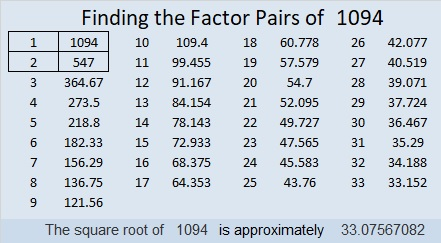# 1094 and Level 6

Should you choose 4 or 8 as the common factor of 32 and 16 in this puzzle?
Is 3 or 9 the common factor needed for 9 and 18?
And is 4 or 6 the common factor for 36 and 12 that will make this puzzle work?
In each of those cases, only one of those factors will work. Which one will it be?

The other clues will help you know where to logically start this puzzle. There is no need to guess and check. The entire puzzle can be solved using logic. Have fun!Print the puzzles or type the solution in this excel file: 10-factors-1087-1094

Now I’ll tell you something about the number 1094:

• 1094 is a composite number.
• Prime factorization: 1094 = 2 × 547
• The exponents in the prime factorization are 1 and 1. Adding one to each and multiplying we get (1 + 1)(1 + 1) = 2 × 2 = 4. Therefore 1094 has exactly 4 factors.
• Factors of 1094: 1, 2, 547, 1094
• Factor pairs: 1094 = 1 × 1094 or 2 × 547
• 1094 has no square factors that allow its square root to be simplified. √1094 ≈ 33.075671094 is palindrome 2A2 in BASE 21 (A is 10 base 10) because 2(21²) + 10(21) + 2(1) = 1094

This site uses Akismet to reduce spam. Learn how your comment data is processed.